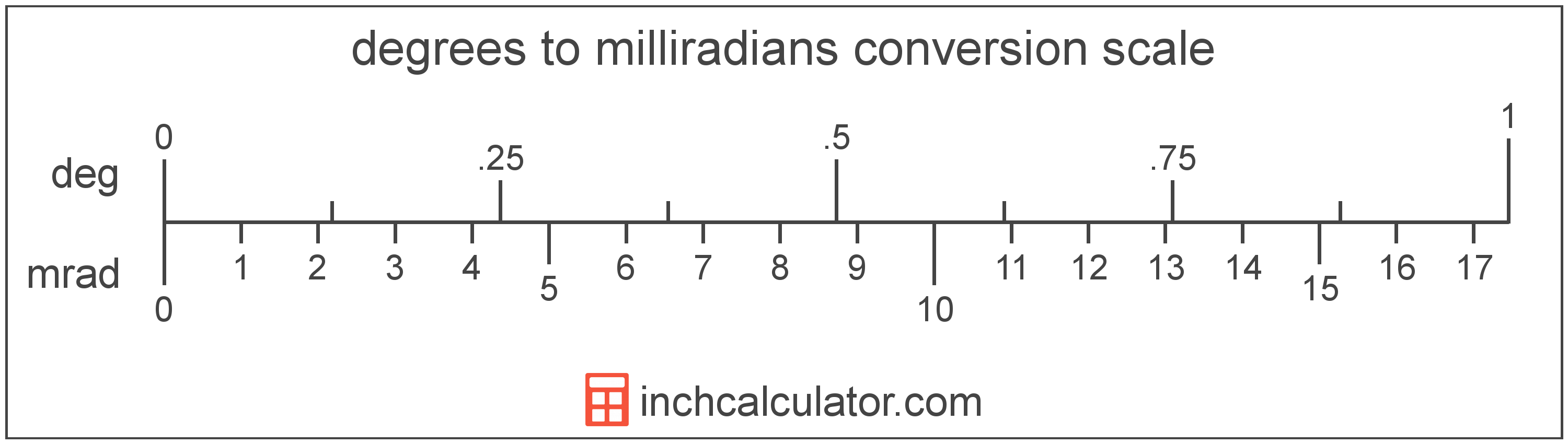Enter the angle in degrees below to get the value converted to milliradians.Do you want to convert milliradians to degrees?

## How to Convert Degrees to Milliradians

To convert a measurement in degrees to a measurement in milliradians, multiply the angle by the following conversion ratio: 17.453293 milliradians/degree.

Since one degree is equal to 17.453293 milliradians, you can use this simple formula to convert:

The angle in milliradians is equal to the angle in degrees multiplied by 17.453293.

For example, here's how to convert 5 degrees to milliradians using the formula above.### How Many Milliradians Are in a Degree?

There are 17.453293 milliradians in a degree, which is why we use this value in the formula above.

## What is a Degree?

A degree is a measure of angle equal to 1/360th of a revolution, or circle. The number 360 has 24 divisors, making it a fairly easy number to work with. There are also 360 days in the Persian calendar year, and many theorize that early astronomers used 1 degree per day.

The degree is an SI accepted unit for angle for use with the metric system. A degree is sometimes also referred to as a degree of arc, arc degree, or arcdegree. Degrees can be abbreviated as °, and are also sometimes abbreviated as deg. For example, 1 degree can be written as 1° or 1 deg.

Degrees can also be expressed using arcminutes and arcseconds as an alternative to using the decimal form. Arcminutes and arcseconds are expressed using the prime (′) and double-prime (″) characters, respectively, although a single-quote and double-quote are often used for convenience.

One arcminute is equal to 1/60th of a degree, and one arcsecond is equal to 1/60th of an arcminute.

Protractors are commonly used to measure angles in degrees. They are semi-circle or full-circle devices with degree markings allowing a user to measure an angle in degrees. Learn more about how to use a protractor or download a printable protractor.

A milliradian is equal to 1/1,000 of a radian. One milliradian is equal to 0.0573 degrees and there are 6,283 milliradians in a full revolution/circle. The milliradian is also a commonly used unit in mathematics.

The milliradian is a multiple of the radian, which is the SI derived unit for angle. In the metric system, "milli" is the prefix for thousandths, or 10-3. A milliradian is sometimes also referred to as a mil. Milliradians can be abbreviated as mrad; for example, 1 milliradian can be written as 1 mrad.

## Degree to Milliradian Conversion Table

Table showing various degree measurements converted to milliradians.